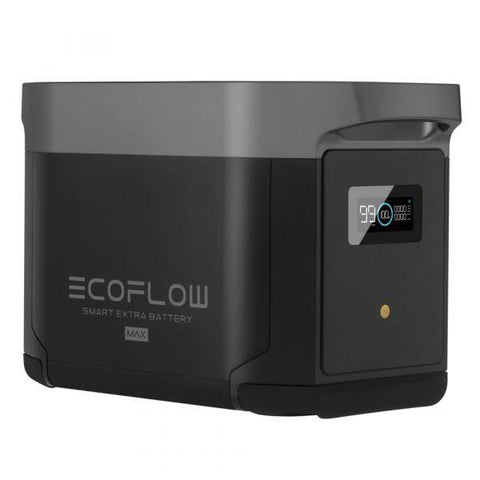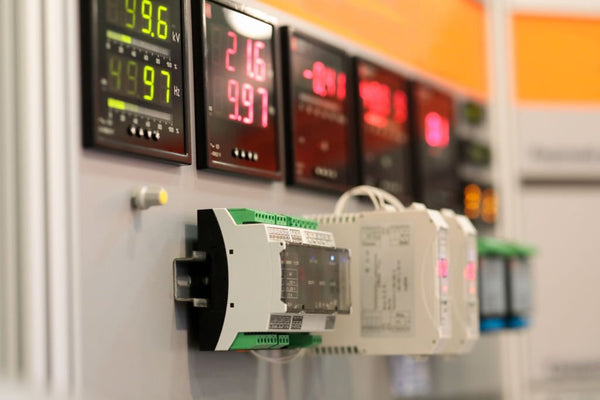Watts to VA Calculator | How to Convert & Why You Should - ShopSolar.com

# Watts to VA Calculator

As many of us are doing more solar projects at home, it’s become important to know how to convert particular units of measurement into others.

This makes things easier when trying to figure out how much power you need for your home or cabin compared to how much power your solar project can generate, and how much the internal circuitry can handle.

In this article, we’ll be covering watt-to-volt-ampere conversion, why it's necessary, and how to do it.

## Convert Watts to VA

Watt is the unit of measurement we assign to what’s known as real power. At the same time, volt-ampere is the unit for apparent power.Watts and volt-amperes are different when it comes to either DC or AC circuits. In a DC circuit, which is found in most 200-watt 12-volt solar panels,  watts and volt-amperes are equal because of the DC circuit’s power factor, which is unity. This can be expressed in the following mathematical equation:

Pdc = VA = Vdc 🗙 Idc

This is more complicated when it comes to an AC circuit, where the power factor determines the apparent and real power. An AC circuit is often part of an off-grid solar power system as an inverter that converts DC to AC.

An AC circuit uses a mathematical expression to determine the relationship between the real power and apparent power. The expression looks like this:

Apparent Power (S) or VA = Real Power (P) or Watt / Power Factor (Pf) or VA = Watt / Pf

### What Do We Use It for?

Determining the amount of volt-ampere for apparent power is often used to simplify calculations and power ratings of currents drawn in a UPS, like the EcoFlow Delta MaxThe value also helps us to determine what kind of circuit breaker or power supply is needed for electronic devices or anything electrical, like computers and other peripherals. To avoid any issues if an electrical surge were to happen, the power supply should always have a higher rating than the equipment.

Volt-amperes are also useful to calculate reactive power. Capacitors and inductors need reactive power to create electric and magnetic fields. The measurement is referred to as volt-ampere reactive and is typically used for power transmission lines.

## Other Types of Watt Conversions

In addition to converting watts into volt-amperes, there are other kinds of watt conversions that are used for a variety of purposes. Below are a few conversions, how to calculate them, and what they’re often used for.

### Watt-hours (Wh) to Milliamp-hours (mAh)

You’ll often use watt-hours and milliampere-hours to describe battery capacity. Sometimes this requires you to convert watt-hours to milliamp-hours

Watt-hours measure the amount of energy going to the load. It’s the total amount of power the battery is able to supply for one hour. Milliamp-hours means how long a battery can supply one milliampere of current, as well as the maximum amount of current for one hour.

A 10mAh battery can thus supply a load of 10mA for an hour before losing its charge, or 1 milliampere for ten hours if the load’s capacity is 1A.

Converting Watt-hours to milliamp-hours can be done using the following equation:

Q (mAh) = E(Wh) 🗙 1 000 / V(v)

### Watts to Amp-hours (Ah)

We convert watt-hours to amp-hours to make comparing things like battery capacities. Since a few brands have started labeling their batteries in Watt-hours, especially ones for solar panels.It’s also helpful for designing an electrical system powered by solar since wire gauge, fuse sizes, and max charge and discharge rates all use amperage instead of wattage.

To find the amp-hours, you first have to convert watts into watt-hours. Watt-hours can be calculated by multiplying the battery voltage by the number of Amps. For example, a 12 V 200 Ah battery (12 Volts with a capacity of 200Ah) is equal to 2,400 Wh.

Converting the Watt-hours into Amp-hours is easy enough. Just divide the Watt-hours by the number of Volts, and there you go.

### Direct Current To Alternate Current

To get AC watts, you have to multiply the performance test conditions (PTC) of the DC wattage by the inverter’s efficiency. Most inverters’ efficiencies run around 95%, so the DC rating has to be multiplied by 0.95. That, or you could use a DC watts to AC watts conversion calculator to make things a lot easier.

## DC Circuits & AC Circuits

As briefly mentioned when it comes to DC and AC circuits; volt-amperes and watts can mean different things depending on the electrical circuit.

In a DC circuit, the power in watts is equal to the voltage and current. When these circuits have more than one device connected to them, the power rating of those devices can be added linearly, which adds up to the total amount of power the circuit would need to function.An AC circuit acts differently. Here, power refers to instant power and it can change after a certain amount of time has passed.

The average power value is calculated by combining multiple values of instant power over a certain period of time and is then divided by that same time frame. The result is expressed as watts in the AC circuit with voltage going across it, current going through it, and for how long (time period).

### AC Circuit Reactance

Power and volt-amperes are equal to each other only when the circuit has no reactance. Reactance is introduced when an inductor or capacitor is involved in the circuit.

Most AC circuits have reactance, which means the volt-amperes are more than the delivered power in watts. This is why watts often refer to real power and volt-amperes refer to apparent power.

Did You Find Our Blog Helpful? Then Consider Checking: